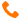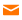+91-9999-283-283/9540-283-283info@sisoft.in```Hints: Swapping two numbers without using temp variable in Java with bitwise operator Bitwise operators can also be used to swap two numbers without using third variable. XOR bitwise operator returns zero if both operand is same i.e. either 0 or 1 and returns 1 if both operands are different e.g. one operand is zero and other is one. By leveraging this property, we can swap two numbers in Java. Here is code example of swapping two numbers without using temp variable in Java using XOR bitwise operand: A B A^B (A XOR B) 0 0 0 (zero because operands are same) 0 1 1 1 0 1 (one because operands are different) 1 1 0 int a = 2; //0010 in binary int b = 4; //0100 in binary System.out.println("value of a and b before swapping, a: " + a +" b: " + b); //swapping value of two numbers without using temp variable and XOR bitwise operator a = a^b; //now a is 6 and b is 4 b = a^b; //now a is 6 but b is 2 (original value of a) a = a^b; //now a is 4 and b is 2, numbers are swapped System.out.println("value of a and b after swapping using XOR bitwise operation, a: " + a +" b: " + b); value of a and b before swapping, a: 2 b: 4 value of a and b after swapping using XOR bitwise operation, a: 4 b: 2 ============================================================================ Shift left multiplies by 2; shift right divides by 2 int a=8 ; System.out.println(a<<2) ; System.out.println(a>>2) ; ============================================================ Using bitwise ooperator to find out odd and even numbers AND (&) operator returns a one in each bit position for which the corresponding bits of both operands are ones; otherwise returns zero. The idea to find odd or even using the last digit of a number will also work for the numbers in binary format. The binary number is odd if its last digit is 1 and even if its last digit is 0. To get the last digit of the binary number, do AND (&) operation of the number with 1. number & 1 will give you the last digit of the binary number. For example , 5 is odd, let us find out using bitwise AND(&) operation 0000 0101 & 0000 0001 = 0000 0001, as the last digit of the binary number is 1 , then it is odd. Number 6 is even , let us see using bitwise AND (&) operation 0000 0110 & 0000 0001 = 0000 0000, as the last digit is 0 , then it is even. The line of code in java using Bitwise AND(&) operation is if( (number&1) == 0) sop(“even”) else sop (“odd”); ==================================================================== ```